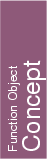## Kernel::ConstructVector_2

A model for this must provide:

Kernel::Vector_2
 fo.operator() ( Kernel::Point_2 a, Kernel::Point_2 b)
introduces the vector b-a.

Kernel::Vector_2 fo.operator() ( Origin o, Kernel::Point_2 b)
introduces the vector b.

Kernel::Vector_2 fo.operator() ( Kernel::Point_2 a, Origin o)
introduces the vector -a.

Kernel::Vector_2 fo.operator() ( Kernel::Segment_2 s)
introduces the vector s.target()-s.source().

Kernel::Vector_2 fo.operator() ( Kernel::Ray_2 r)
introduces a vector having the same direction as r.

Kernel::Vector_2 fo.operator() ( Kernel::Line_2 l)
introduces a vector having the same direction as l.

Kernel::Vector_2 fo.operator() ( Null_vector NULL_VECTOR)
introduces a null vector .

### Refines

AdaptableFunctor (with two arguments)